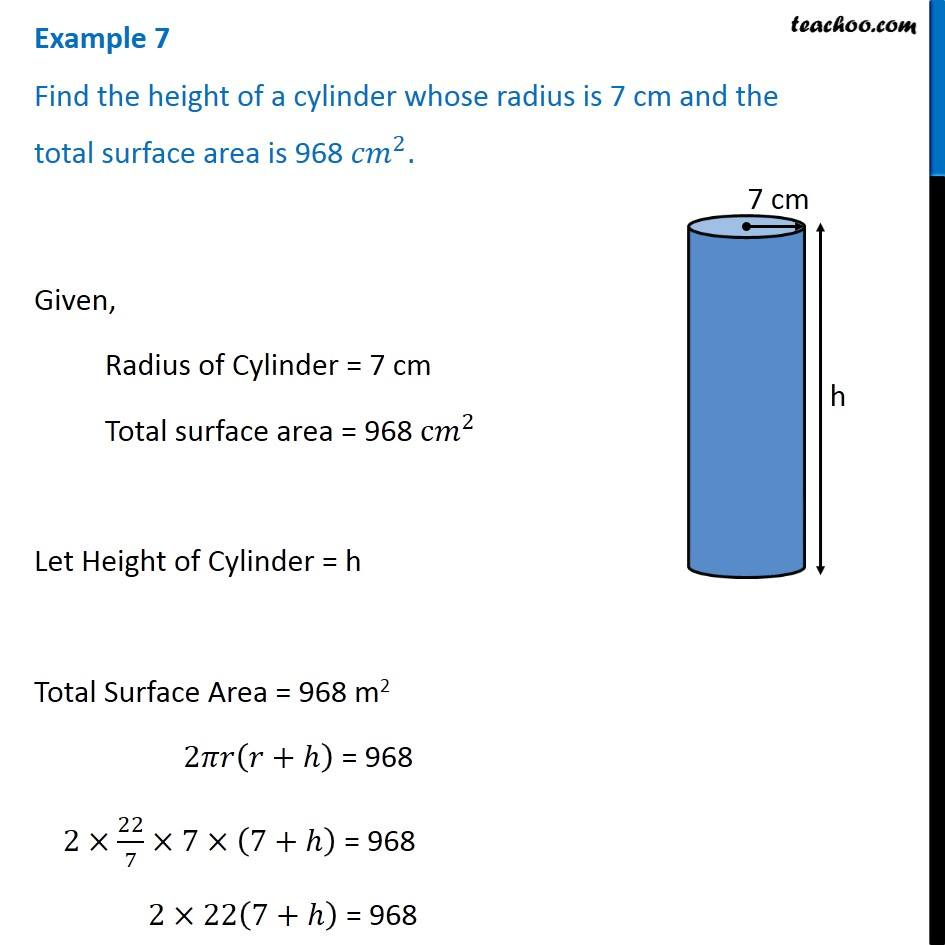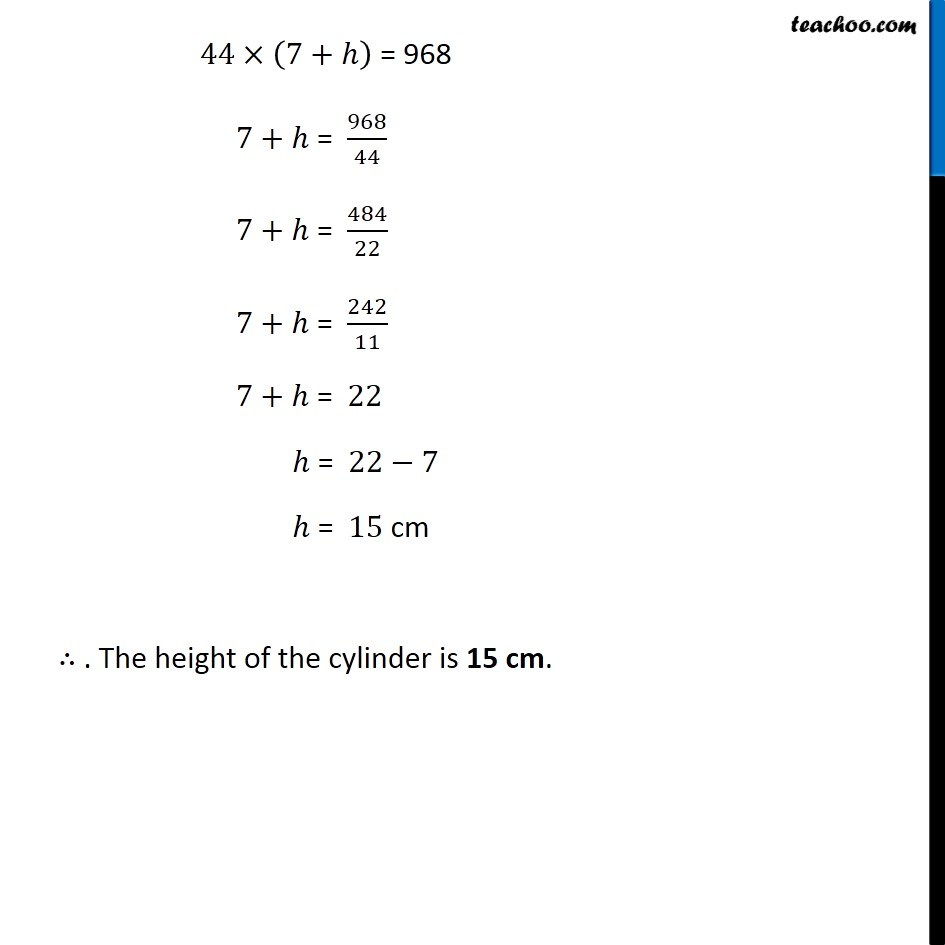Examples

Chapter 9 Class 8 Mensuration
Serial order wiseLearn in your speed, with individual attention - Teachoo Maths 1-on-1 Class

### Transcript

Example 7 Find the height of a cylinder whose radius is 7 cm and the total surface area is 968 〖𝑐𝑚〗^2. Given, Radius of Cylinder = 7 cm Total surface area = 968 c𝑚^2 Let Height of Cylinder = h Total Surface Area = 968 m2 2𝜋𝑟(𝑟+ℎ) = 968 2×22/7×7×(7+ℎ) = 968 2×22(7+ℎ) = 968 44×(7+ℎ) = 968 7+ℎ = 968/44 7+ℎ = 484/22 7+ℎ = 242/11 7+ℎ = 22 ℎ = 22−7 ℎ = 15 cm ∴ . The height of the cylinder is 15 cm.